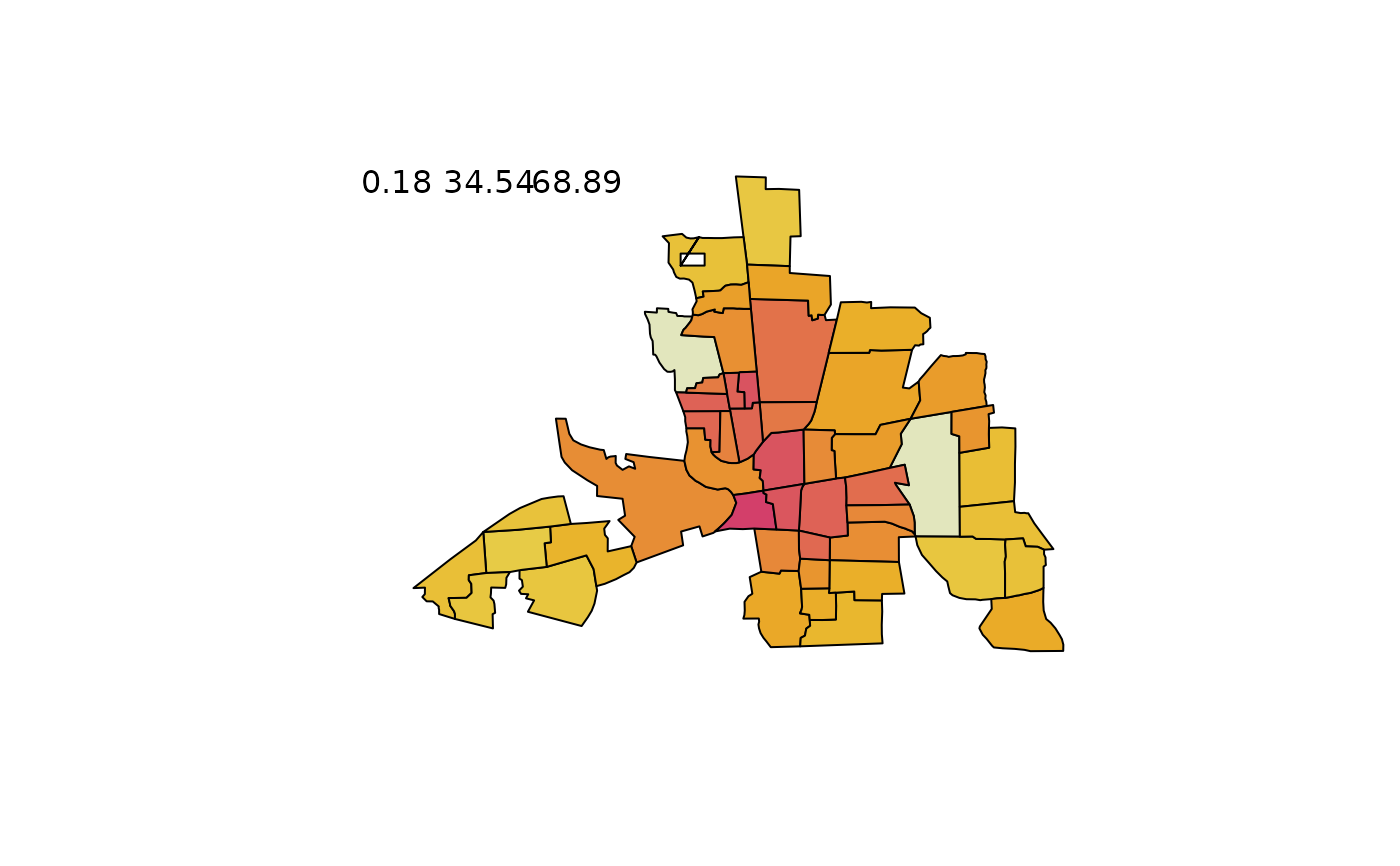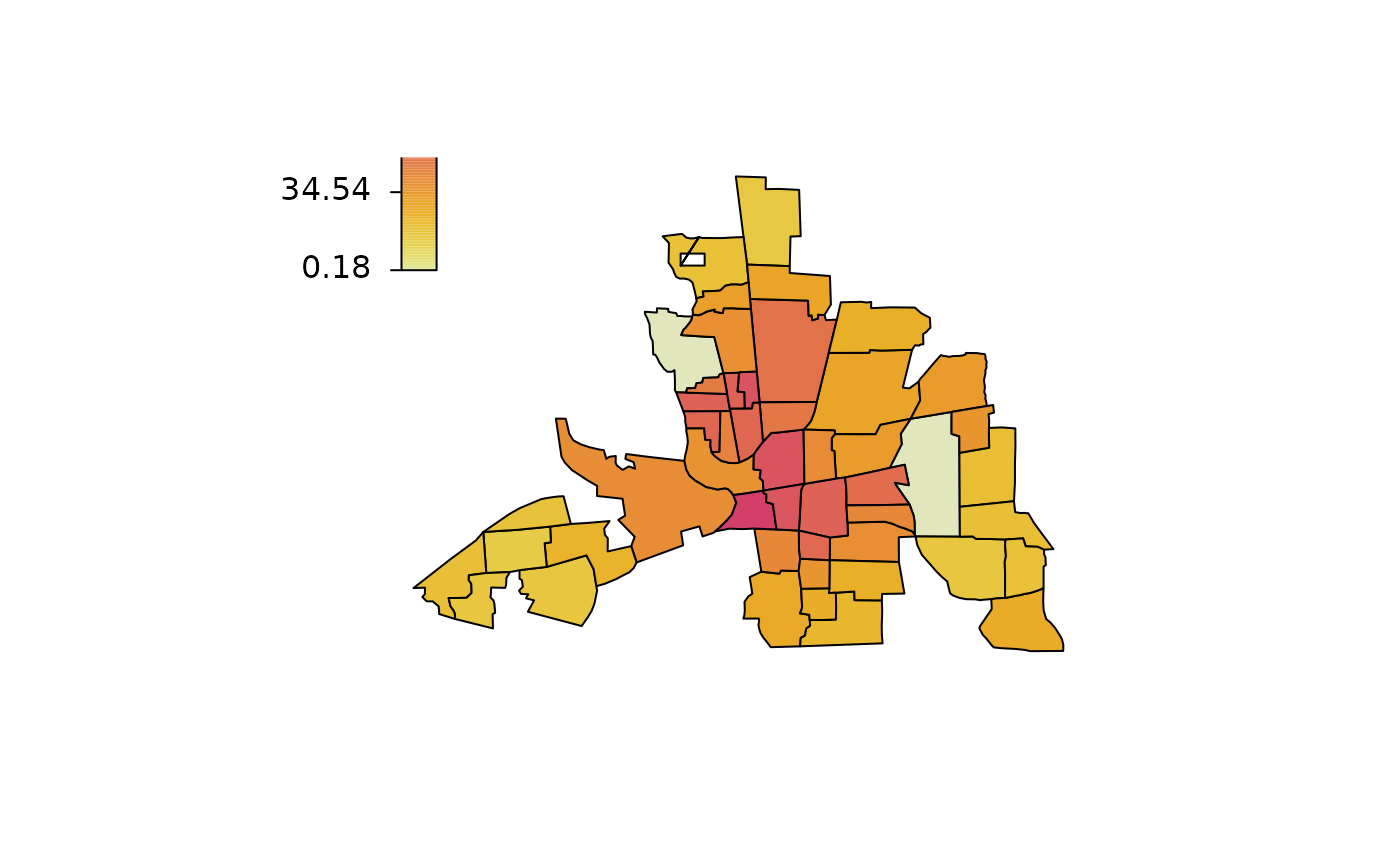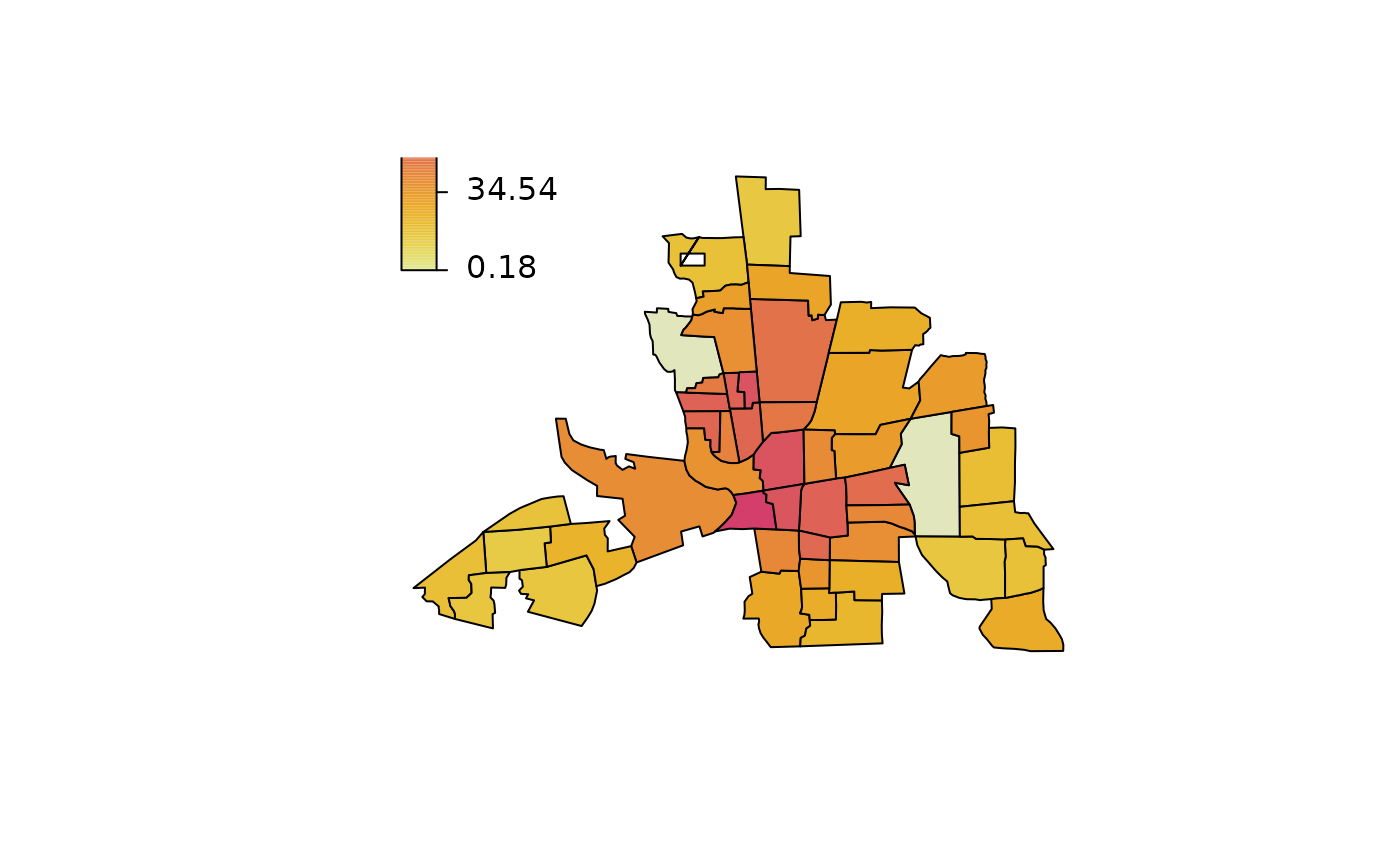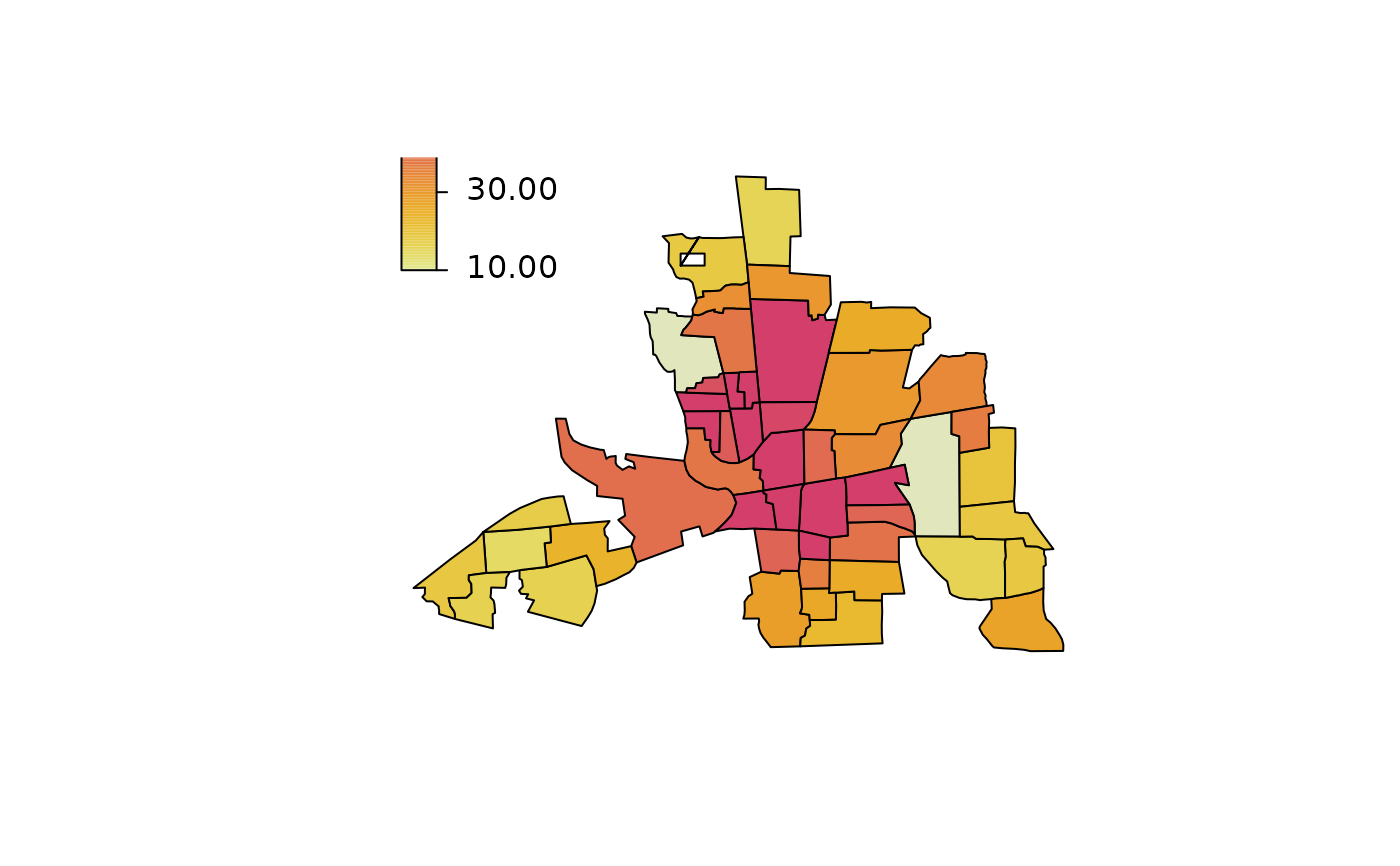The function takes a list polygons and draws the corresponding map. Different colors for each polygon can be used.

plotmap(map, x = NA, id = NULL, select = NULL,
legend = TRUE, names = FALSE, values = FALSE, ...)

## Arguments

map

The map to be plotted, usually an object that inherits from SpatialPolygons, but may also be a list of polygons, i.e., each list entry is a matrix with x- and y-coordinates.

x

A vector, data.frame or matrix. In the latter case x should have two columns, one column that is the region identifier and one that contains the values to be plotted. In case x is a data.frame, the function searches for "character" or "factor" columns for the region identifier. If x is a matrix, the second column of x is supposed to be the region identifier. If x = NA and map is an object of class "SpatialPolygonsDataFrame" only the polygons will be plotted without using the data.

id

If argument x is a vector, argument id should contain the region identifier vector.

select

Select the column of the data in x which should be used for plotting, may be an integer or character with the corresponding column name.

legend

Should a color legend be added to the plot, see also function colorlegend.

names

If set to TRUE the name for each polygon will also be plotted at the centroids of the corresponding polygons.

values

If set to TRUE the corresponding values for each polygon will also be plotted at the centroids of the polygons.

...

Arguments to be passed to colorlegend and others, e.g. change the border of the polygons and density (mdensity for missing regions in id), see polygon.

plot2d, plot3d, sliceplot, plotblock.

## Examples

## Example from mgcv ?mrf.
## Load Columbus Ohio crime data (see ?columbus for details and credits).
data("columb", package = "mgcv")
data("columb.polys", package = "mgcv")

## Plot the map.
plotmap(columb.polys)## Plot aggregated data.
a <- with(columb, aggregate(crime,
by = list("district" = district), FUN = mean))

plotmap(columb.polys, x = a$x, id = a$district)plotmap(columb.polys, x = a$x, id = a$district,
pos = "topleft")
plotmap(columb.polys, x = a$x, id = a$district,
pos = "topleft", side.legend = 2)plotmap(columb.polys, x = a$x, id = a$district,
pos = "topleft", side.legend = 2, side.ticks = 2)plotmap(columb.polys, x = a$x, id = a$district,
pos = "topleft", side.legend = 2, side.ticks = 2,
col = heat_hcl, swap = TRUE)
plotmap(columb.polys, x = a$x, id = a$district,
pos = "topleft", side.legend = 2, side.ticks = 2,
col = heat_hcl, swap = TRUE, range = c(10, 50))plotmap(columb.polys, x = a$x, id = a$district,
pos = "topleft", side.legend = 2, side.ticks = 2,
col = heat_hcl(5), swap = TRUE, range = c(10, 50),
lrange = c(0, 60))